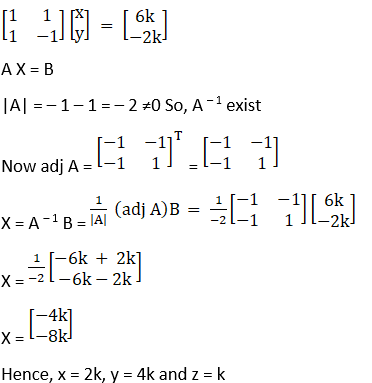# RD Sharma Solutions For Class 12 Maths Exercise 8.2 Chapter 8 Solution of Simultaneous Linear Equations

RD Sharma Solutions for Class 12 Maths Exercise 8.2 Chapter 8 Solution of Simultaneous Linear Equations consists of problems on finding the homogeneous system of linear equations. These problems are solved by BYJU’S experts in a simple way to speed up the exam preparation of students. It mainly helps during revision and improves confidence among students before appearing for the board exam.

To gain better knowledge about the concepts explained in this exercise, they can refer RD Sharma Solutions Class 12 Maths Chapter 8 Solution of Simultaneous Linear Equations Exercise 8.2 free PDF, which are given below.

## RD Sharma Solutions For Class 12 Chapter 8 Solution of Simultaneous Linear Equations Exercise 8.2:-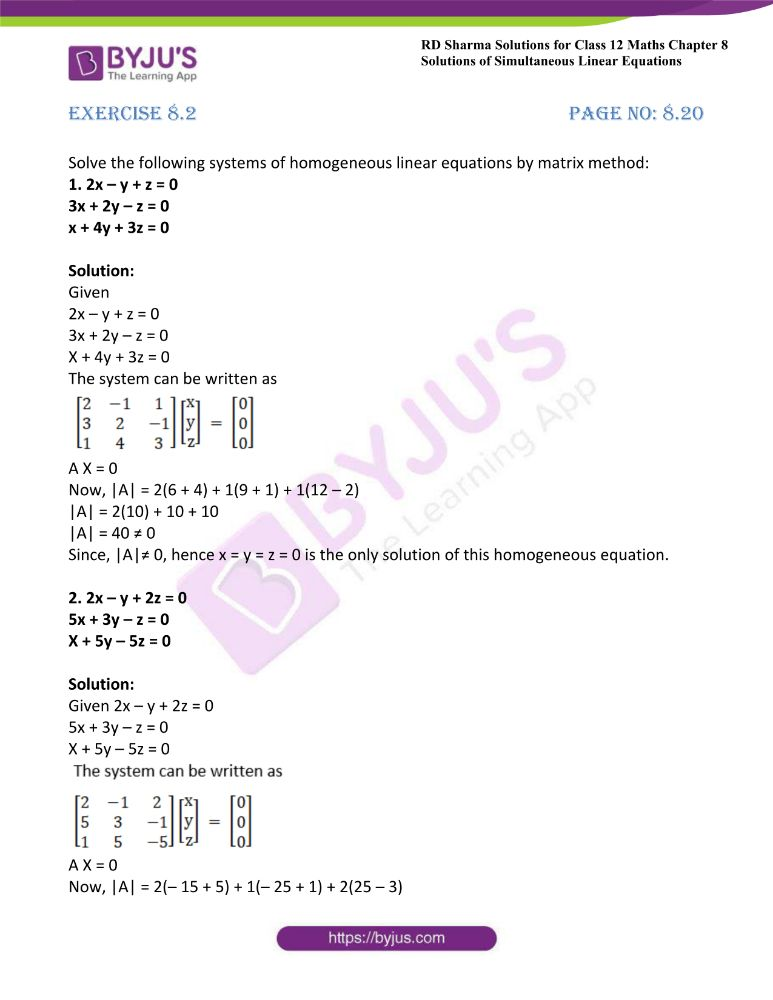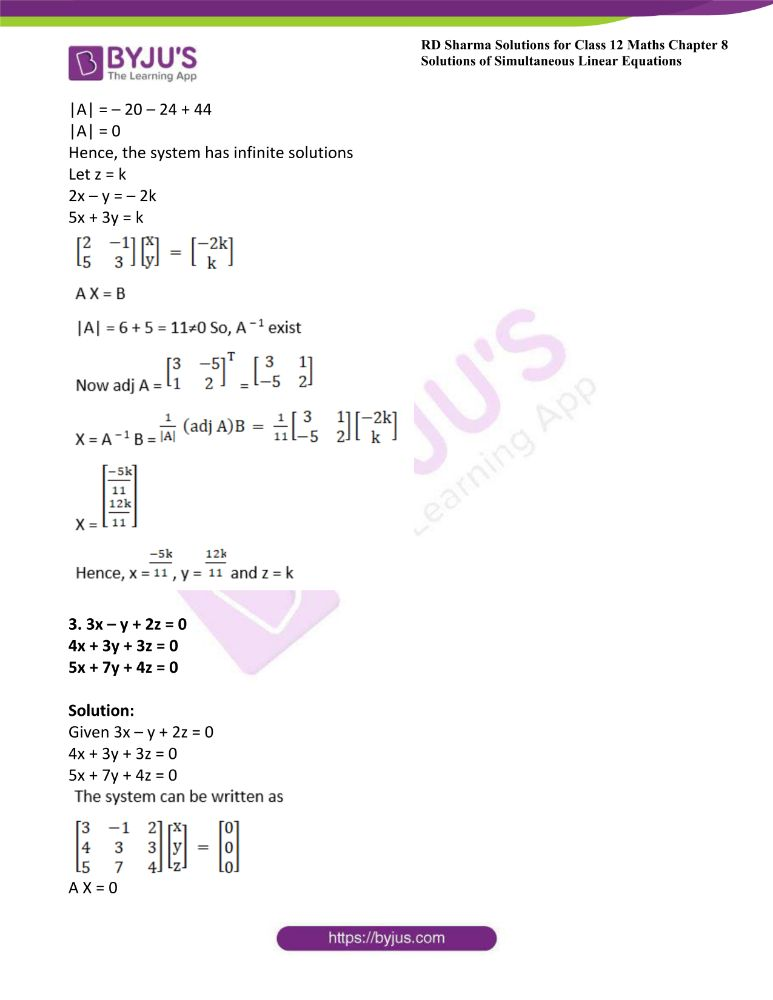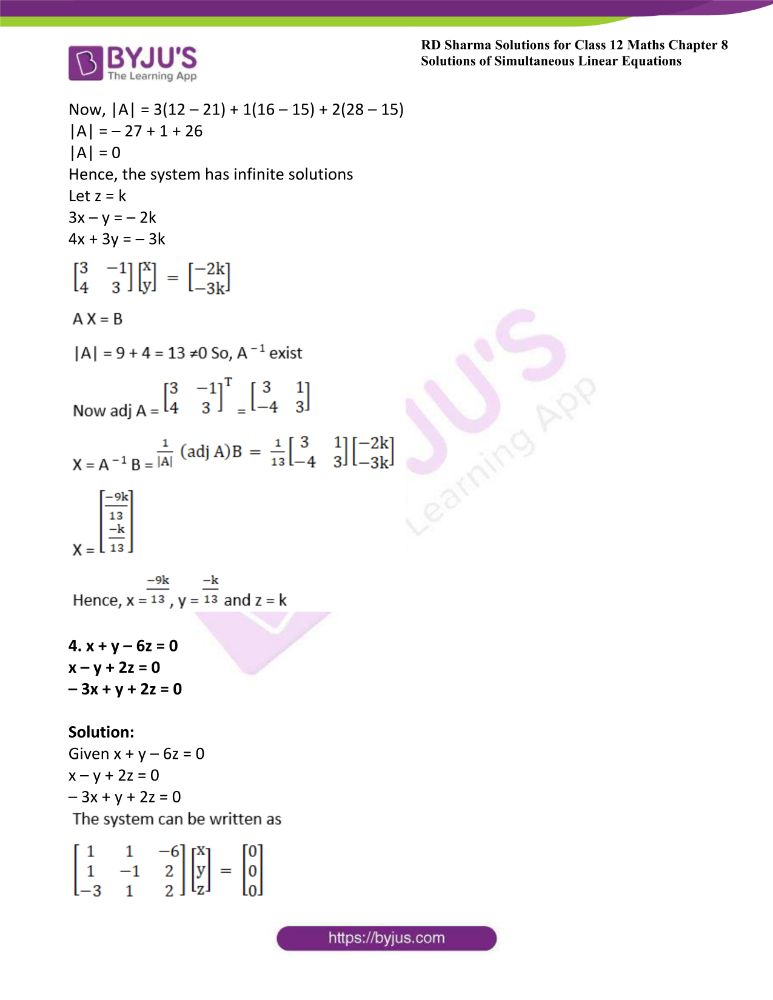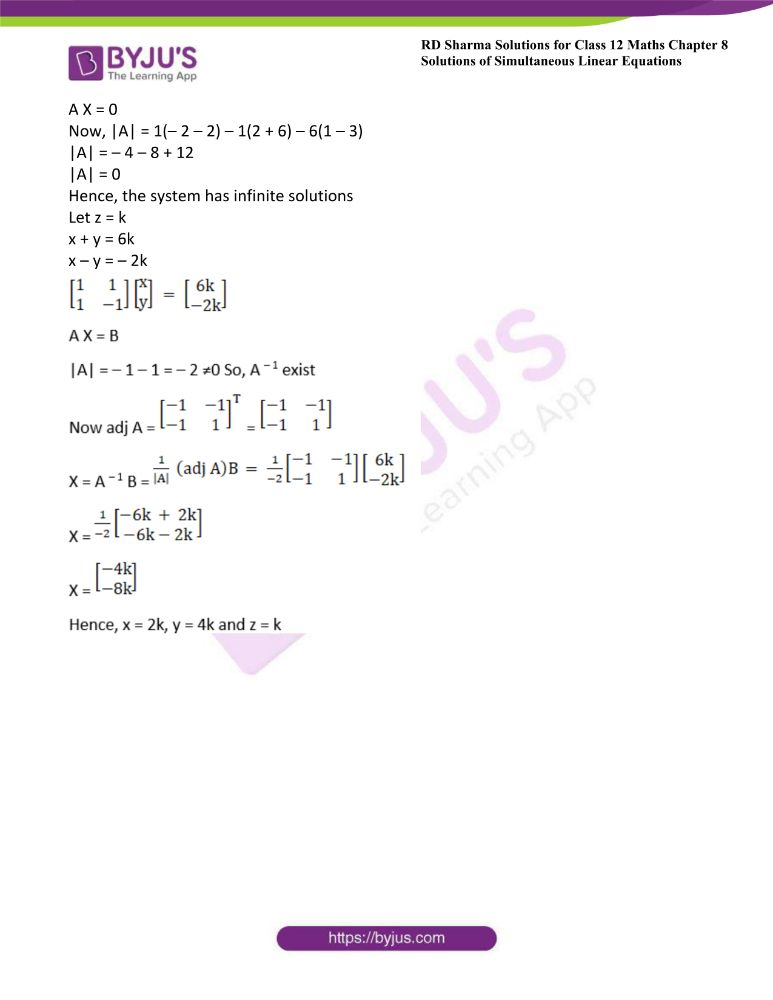### Access another exercise of RD Sharma Solutions For Class 12 Chapter 8 – Solution of Simultaneous Linear Equations

Exercise 8.1 Solutions

### Access answers to Maths RD Sharma Solutions For Class 12 Chapter 8 – Solution of Simultaneous Linear Equations Exercise 8.2

Exercise 8.2 Page No: 8.20

Solve the following systems of homogeneous linear equations by matrix method:

1. 2x – y + z = 0

3x + 2y – z = 0

x + 4y + 3z = 0

Solution:

Given

2x – y + z = 0

3x + 2y – z = 0

X + 4y + 3z = 0

The system can be written as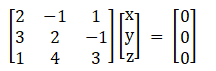A X = 0

Now, |A| = 2(6 + 4) + 1(9 + 1) + 1(12 – 2)

|A| = 2(10) + 10 + 10

|A| = 40 ≠ 0

Since, |A|≠ 0, hence x = y = z = 0 is the only solution of this homogeneous equation.

2. 2x – y + 2z = 0
5x + 3y – z = 0
X + 5y – 5z = 0

Solution:

Given 2x – y + 2z = 0

5x + 3y – z = 0

X + 5y – 5z = 0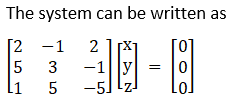A X = 0

Now, |A| = 2(– 15 + 5) + 1(– 25 + 1) + 2(25 – 3)

|A| = – 20 – 24 + 44

|A| = 0

Hence, the system has infinite solutions

Let z = k

2x – y = – 2k

5x + 3y = k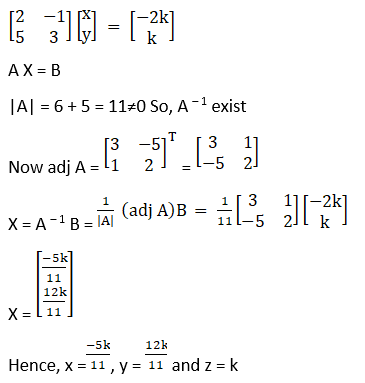3. 3x – y + 2z = 0
4x + 3y + 3z = 0
5x + 7y + 4z = 0

Solution:

Given 3x – y + 2z = 0

4x + 3y + 3z = 0

5x + 7y + 4z = 0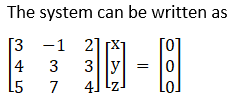A X = 0

Now, |A| = 3(12 – 21) + 1(16 – 15) + 2(28 – 15)

|A| = – 27 + 1 + 26

|A| = 0

Hence, the system has infinite solutions

Let z = k

3x – y = – 2k

4x + 3y = – 3k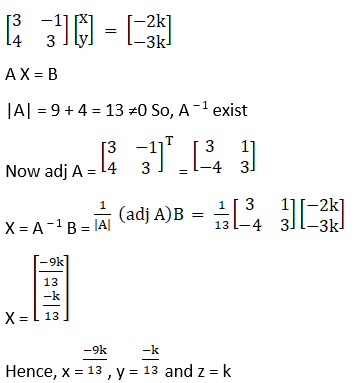4. x + y – 6z = 0
x – y + 2z = 0
– 3x + y + 2z = 0

Solution:

Given x + y – 6z = 0

x – y + 2z = 0

– 3x + y + 2z = 0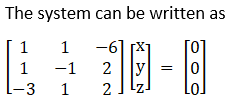A X = 0

Now, |A| = 1(– 2 – 2) – 1(2 + 6) – 6(1 – 3)

|A| = – 4 – 8 + 12

|A| = 0

Hence, the system has infinite solutions

Let z = k

x + y = 6k

x – y = – 2k